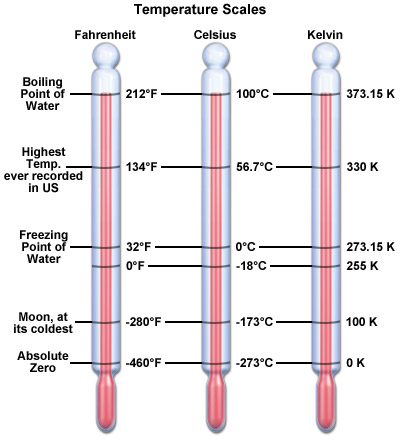# Difference Between Thermal Energy and Temperature

There are a lot of differences between temperature and thermal energy. Temperature is a parameter which can be directly measured with the help of various devices such as thermometers, whereas thermal energy can be calculated. For example, nobody can predict the amount of thermal energy in a black box, using a thermometer.

The thermal energy of the system is always positive. The temperature on the other hand can be negative in all the temperature measurement systems like Centigrade and Fahrenheit, except for the absolute Kelvin system, which consider 0K at -273 degree Centigrade. This also explains that both temperature and thermal energy have separate units – thermal energy is measured in Joules (J) whereas temperature can be measured in Centigrade, Fahrenheit or Kelvin. There are many other scales as well but the mentioned three are mostly used.

Thermal energy can be transferred from one state to another without the change in temperature.

### Instructions

• 1

Thermal energy

Thermal energy is basically heat and is measured in Joules. This can also be referred to as the internal energy of the system. The thermal energy in a system is produced by the movement of molecules, atom and electrons. These particles have kinetic energy, which increases when they collide with each other. This results in the release of photons, which increase the heat content, and thus the temperature of the system.

Thermal energy determines the randomness of the system. Higher the thermal energy, greater will be the randomness, meaning the atoms can move more freely. This form of energy can be converted into other forms; the most notable example in this case is that of heat engine, where heat energy is converted into mechanical energy. In this concept, heat released by the combustion of air with fuel inside the combustion chamber is used to run the turbine, which then runs the shaft.

Thermal energy depends upon the temperature of the object, its mass and its capacity to absorb heat.

Image courtesy: navigantresearch.com• 2

Temperature

Temperature is a measurable property and can be measured using a variety of scales. The SI unit for the measurement of temperature is Kelvin. The absolute zero starts from -273 degree centigrade – below which temperature cannot fall.

The thermal energy of a system is also zero at this absolute temperature.

Image courtesy: nc-climate.ncsu.edu•